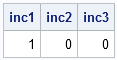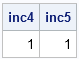The other day I needed to check that a sequence of numerical values was in strictly increasing order. My first thought was to sort the values and compare the sorted and original values, but I quickly discarded that approach because it does not detect duplicate values in a montonic (nondecreasing) sequence.

Instead, I decided to use the UNIQUE function in the SAS/IML language, which returns a sorted vector of the input values and removes duplicate values. The following PROC IML statements define a module that tests whether the row vector x contains strictly increasing values:

```proc iml; /** Test whether the row vector, x, is strictly increasing **/ start TestIncreasing(x); u = unique(x); if ncol(u) ^= ncol(x) then return( 0 ); if any(u ^= x) then return( 0 ); return( 1 ); finish;```

The module consists of the following steps:

1. Use the UNIQUE function to create a sorted increasing list of the unique values of x. The remainder of the module tests whether u and x contain the same values in the same order.
2. If the number of columns in u is different than the number of columns of x, then there must have been a repeated value in x that was removed by the UNIQUE function. Return 0 (failure) in this case.
3. Do an elementwise comparison of the value in u and x. If any of the values are different (as determined by the ANY function), return 0. (In the second chapter (p. 38-39) of my book, Statistical Programming with SAS/IML Software, I explain why you need to use the ANY function in this case.) Notice that you do not need to write a loop in order to test every value.
4. If the vector x passes both of those tests, it is in increasing order. Return 1 for success.

You can test the module on a few examples. Only the first example is an increasing sequence:

```inc1 = TestIncreasing( 1:10 ); inc2 = TestIncreasing( {0 1 1 2 } ); inc3 = TestIncreasing( {0 1 2 -1} ); print inc1 inc2 inc3;```### Generalizing the Module

I try to write modules that are robust to the shape of the input argument. The module TestIncreasing works correctly for row vectors, but Step 2 (the first IF-THEN statement) will return 0 (failure) if the input argument is not a row vector.

There is an easy way to accomodate input arguments that are not necessarily row vectors: use the ROWVEC function module, which part of the IMLMLIB module library.

Change the beginning of the module to the following statements:

```start TestIncreasing(_x); x = rowvec(_x); /** ...continue with the module definition... **/```

With that small change, the module can be used to detect whether a column vector has values in increasing order, and even whether a matrix of values (considered in row-major order) is in increasing order:

```inc4 = TestIncreasing( {0,4,6,9} ); inc5 = TestIncreasing( {0 1, 3 4, 7 9} ); print inc4 inc5;```### An easier method

Update 14AUG2014: I'm not sure why I wrote such a complicated module. Here's an easier approach that uses the DIF function, which was introduced in SAS/IML 9.22:

```start TestIncreasing(x); d = dif(shape(x,0,1)); return( all(d[2:nrow(d)]> 0) ); finish;```
ShareDistinguished Researcher in Computational Statistics

Rick Wicklin, PhD, is a distinguished researcher in computational statistics at SAS and is a principal developer of SAS/IML software. His areas of expertise include computational statistics, simulation, statistical graphics, and modern methods in statistical data analysis. Rick is author of the books Statistical Programming with SAS/IML Software and Simulating Data with SAS.

1.2.